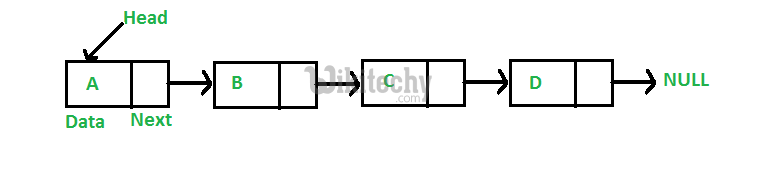# C Algorithm – Introduction for Linked List | Set 1

C Algorithm - Introduction for Linked List | Set 1 - Linked List - Like arrays, Linked List is a linear data structure. Unlike arrays

Like arrays, Linked List is a linear data structure. Unlike arrays, linked list elements are not stored at contiguous location; the elements are linked using pointers.Arrays can be used to store linear data of similar types, but arrays have following limitations.
1) The size of the arrays is fixed: So we must know the upper limit on the number of elements in advance. Also, generally, the allocated memory is equal to the upper limit irrespective of the usage.
2) Inserting a new element in an array of elements is expensive, because room has to be created for the new elements and to create room existing elements have to shifted.

For example, in a system if we maintain a sorted list of IDs in an array id[].

id[] = [1000, 1010, 1050, 2000, 2040].

And if we want to insert a new ID 1005, then to maintain the sorted order, we have to move all the elements after 1000 (excluding 1000).
Deletion is also expensive with arrays until unless some special techniques are used. For example, to delete 1010 in id[], everything after 1010 has to be moved.

1) Dynamic size
2) Ease of insertion/deletion

Drawbacks:
1) Random access is not allowed. We have to access elements sequentially starting from the first node. So we cannot do binary search with linked lists.
2) Extra memory space for a pointer is required with each element of the list.

Representation in C:
A linked list is represented by a pointer to the first node of the linked list. The first node is called head. If the linked list is empty, then value of head is NULL.
Each node in a list consists of at least two parts:
1) data
2) pointer to the next node
In C, we can represent a node using structures. Below is an example of a linked list node with an integer data.
In Java, LinkedList can be represented as a class and a Node as a separate class. The LinkedList class contains a reference of Node class type.

C Programming:

``````// A linked list node
struct Node
{
int data;
struct Node *next;
};``````

First Simple Linked List in C Let us create a simple linked list with 3 nodes.

C Programming:

``````// A simple C program to introduce
#include<stdio.h>
#include<stdlib.h>

struct Node
{
int data;
struct Node *next;
};

// Program to create a simple linked
// list with 3 nodes
int main()
{
struct Node* second = NULL;
struct Node* third = NULL;

// allocate 3 nodes in the heap
second = (struct Node*)malloc(sizeof(struct Node));
third = (struct Node*)malloc(sizeof(struct Node));

/* Three blocks have been allocated  dynamically.
We have pointers to these three blocks as first, second and third
|                |               |
|                |               |
+---+-----+     +----+----+     +----+----+
| #  | #  |     | #  | #  |     |  # |  # |
+---+-----+     +----+----+     +----+----+

# represents any random value.
Data is random because we haven’t assigned anything yet  */

head->data = 1; //assign data in first node

/* data has been assigned to data part of first block (block
pointed by head).  And next pointer of first block points to
second.  So they both are linked.

|              |              |
|              |              |
+---+---+     +----+----+     +-----+----+
| 1  | o----->| #  | #  |     |  #  | #  |
+---+---+     +----+----+     +-----+----+
*/

second->data = 2; //assign data to second node
second->next = third; // Link second node with the third node

/* data has been assigned to data part of second block (block pointed by
second). And next pointer of the second block points to third block.
So all three blocks are linked.

|             |             |
|             |             |
+---+---+     +---+---+     +----+----+
| 1  | o----->| 2 | o-----> |  # |  # |
+---+---+     +---+---+     +----+----+      */

third->data = 3; //assign data to third node
third->next = NULL;

/* data has been assigned to data part of third block (block pointed
by third). And next pointer of the third block is made NULL to indicate
that the linked list is terminated here.

|
|
+---+---+     +---+---+       +----+------+
| 1  | o----->|  2  | o-----> |  3 | NULL |
+---+---+     +---+---+       +----+------+

Note that only head is sufficient to represent the whole list.  We can
traverse the complete list by following next pointers.    */

return 0;
}``````

In the previous program, we have created a simple linked list with three nodes. Let us traverse the created list and print the data of each node. For traversal, let us write a general purpose function printList() that prints any given list.

C Programming:

``````// A simple C program for traversal of a linked list
#include<stdio.h>
#include<stdlib.h>

struct Node
{
int data;
struct Node *next;
};

// This function prints contents of linked list starting from
// the given node
void printList(struct Node *n)
{
while (n != NULL)
{
printf(" %d ", n->data);
n = n->next;
}
}

int main()
{
struct Node* second = NULL;
struct Node* third = NULL;

// allocate 3 nodes in the heap
second = (struct Node*)malloc(sizeof(struct Node));
third  = (struct Node*)malloc(sizeof(struct Node));

head->data = 1; //assign data in first node

second->data = 2; //assign data to second node
second->next = third;

third->data = 3; //assign data to third node
third->next = NULL;

return 0;
}``````#### Venkatesan Prabu

Wikitechy Founder, Author, International Speaker, and Job Consultant. My role as the CEO of Wikitechy, I help businesses build their next generation digital platforms and help with their product innovation and growth strategy. I'm a frequent speaker at tech conferences and events.

X